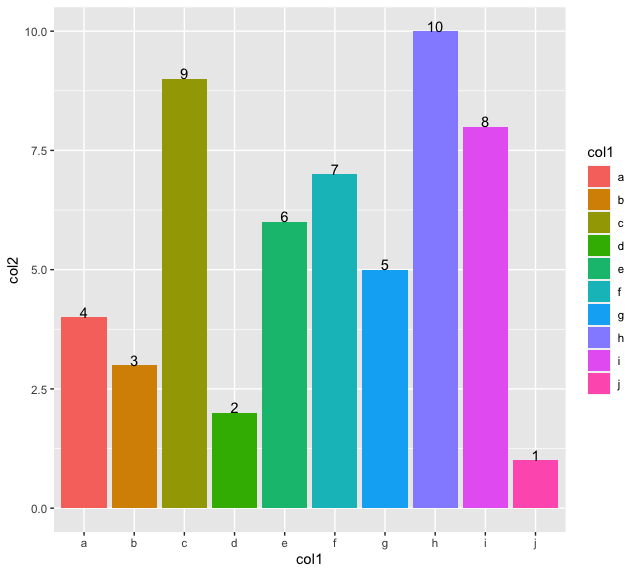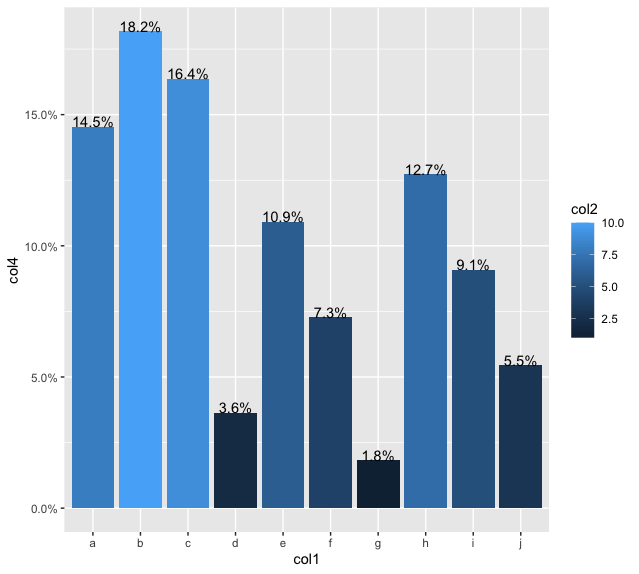Related Articles

# How to add percentage or count labels above percentage bar plot in R?

• Last Updated : 18 Jul, 2021

In this article, we will discuss how to add percentage or count above percentage bar plot in R programming language.

The ggplot() method of this package is used to initialize a ggplot object. It can be used to declare the input data frame for a graphic and can also be used to specify the set of plot aesthetics. The ggplot() function is used to construct the initial plot object and is almost always followed by components to add to the plot.

Syntax:

ggplot(data, mapping = aes())

Parameter :

• data – The data frame used for data plotting
• mapping – Default list of aesthetic mappings to use for plot.

geom_bar() is used to draw a bar plot.

The geom_bar() method is used which plots a number of cases appearing in each group against each bar value. Using the “stat” attribute as “identity” plots and displays the data as it is. The graph can also be annotated with displayed text on the top of the bars to plot the data as it is.

Syntax:

geom_text(aes(label = ), vjust )

The label can be assigned the value of the column to assign the value to each bar of the plot corresponding to each bar value.

Example:

## R

 `library``(``"ggplot"``)`` ` `# creating a data frame``data_frame <- ``data.frame``(col1 = ``sample``(``letters``[1:10]),``                         ``col2 = 1:10,``                         ``col3 = 1)``# printing the data frame``print ``(``"Original DataFrame"``)``print ``(data_frame)`` ` `# plotting a barplot with counts``ggplot``(data_frame, ``aes``(x = col1, y = col2, fill = col1)) + ``  ``geom_bar``(stat = ``"identity"``) +``  ``geom_text``(``aes``(label = col2), vjust = 0)`

Output

``` "Original DataFrame"
col1 col2 col3
1     j    1    1
2     d    2    1
3     b    3    1
4     a    4    1
5     g    5    1
6     e    6    1
7     f    7    1
8     i    8    1
9     c    9    1
10    h   10    1```Similarly, percentages can be added to the plot, but in this case, the legend will be continuous, not discrete.

Example:

## R

 `# importing the required libraries``library``(``"ggplot"``)``library``(``"scales"``)``library``(``"dplyr"``)`` ` `# creating a data frame``data_frame <- ``data.frame``(col1 = ``sample``(``letters``[1:10]),``                         ``col2 = 1:10``                         ``)``# printing the data frame``print ``(``"Original DataFrame"``)``print ``(data_frame)`` ` `# plotting a barplot with percentages``data_frame %>% ``  ``count``(col1 = ``factor``(col1), col2 = col2) %>% ``  ``mutate``(col4 = ``prop.table``(col2)) %>% ``  ``ggplot``(``aes``(x = col1, y = col4, fill = col2, label = scales::``percent``(col4))) + ``  ``geom_col``(position = ``'dodge'``) + ``  ``geom_text``( vjust = 0) + ``  ``scale_y_continuous``(labels = scales::percent)`

Output

``` "Original DataFrame"
col1 col2
1     g    1
2     d    2
3     j    3
4     f    4
5     i    5
6     e    6
7     h    7
8     a    8
9     c    9
10    b   10```My Personal Notes arrow_drop_up